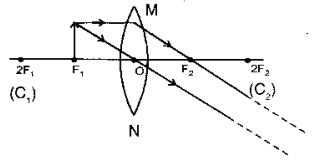# Rohit focused the image of a candle flame on a white screen using a convex lens

Rohit focused the image of a candle flame on a white screen using a convex lens. He noted down the position of the candle, screen and lens as under:
Position of candle = 26.0 cm
Position of convex lens = 50.0 cm
Position of screen = 74.0 cm
(i) What is the focal length of the convex lens?
(ii) Where will the image be formed if he shifts the candle towards the lens at a position of 38 cm?
(iii) Draw a ray diagram to show the formation of the image in case (ii) as said above?

Position of candle = 26 cm
Position of convex lens = 50 cm
Position of screen = 74 cm
Object distance, u = 50-26 = 24 cm
Image distance, v = 74 - 50 = 24 cm
(i) So,
2f = 24 cm
f = 12 cm

(ii) If he shifts the candle towards the lens at a position of 38 cm; then, u = 50 - 38 = 12 cm
which means, that candle is at focus
.’. Image is formed at infinity.

(iii)